# Sums and Correlations

This document defines notation that I use for indicating “summed” values, and also defines a particular type of “correlation” factor that I use. This is an alternative to Averages and Correlations. The two frameworks are equivalent ways of representing the same underlying mathematics.

## Overview

I this section, I’ll try to summarize the minimum you need to know about sums in order to to be able to follow my analyses.

• I denote the sum of a quantity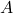by the notation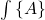.
• In the context of my climate analyses, I use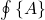to indicate a global average (i.e., an average over the surface of the globe) within some time window.
• I sometimes express things in terms of the weighted average ofrelative to a weight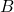, which I denote as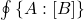.
• I sometimes express things in terms of the multiplicative correlation ofand, which I denote as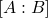. This is a dimensionless quantity. Whenandare uncorrelated,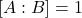. However, in general, it could be larger or smaller than 1. The notation can be extended to multiple variables, as in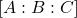, which denotes the correlation of,, and.

These values are related by several identities, which I often use to transform the way a quantity is expressed:

1.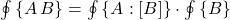2.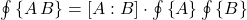3.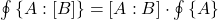(I use the notation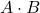to indicate multiplication when I want to make the factors more visually distinct.)

If you are reading one of my analyses, taking in the above information may be enough to allow you to follow the analysis and understand the results. If not, then more detailed information is provided below.

## Sums

### Sum

Ifis a discrete quantity and there are a finite number of instances of, then the summed value of, denoted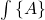, is given by:

(1)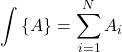Ifis a continuous quantity which varies with respect to a variable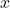, then the value of“summed” overis given by:

(2)Sums are “linear,” meaning they have behavior in a simple way with respect to addition and multiplication by a constant. In particular, if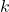is a constant, then:

(3)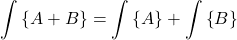(4)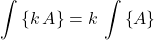### Sum over more than one variable

One can sum over different variables. When I want to distinguish sum with respect to different variables, I denote the sum ofwith relative to the variableby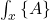. When averaging over multiple variables, it doesn’t matter in which order summing is done:

(5)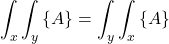### Weighted sum

The weighted sum ofwith respect tois defined by:

(6)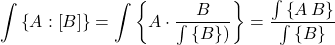When working with averages of quantities that are the result of multiplying several factors together, using weighted sums can make expressions work out more neatly.

Ifhas minimum value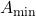and maximum value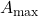, then, providedeverywhere,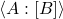will always be between these two limits:

(7)## Multiplicative Correlations

When people talk about the “correlation” of two variables, they are typically talking about what could be referred to as an “additive” correlation. Such a “correlation” ofandinvolves calculating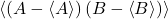where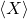denotes the average of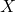. The relationship between each variable’s additive deviation from its mean (average) vale.

Rather than focusing on additive correlations, I’d like to consider each variable’s multiplicative deviation from its mean value. I denote the multiplicative correlation ofand(or the multiplicative correlation factor forand) aswhere this is defined by:

(8)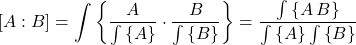This can be generalized to more variables:

(9)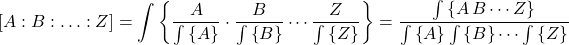Variablesandare said to be “uncorrelated” if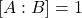.

Writing formulas using multiplicative correlation factors allows one to clearly distinguish what depends on the average values of individual variables, and what depends on relationships between variables (as characterized by their correlation).

If the variablesandsatisfies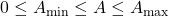and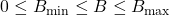, then their multiplicative correlation satisfies:

(10)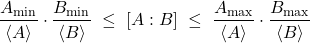Note: The terms I use, “additive correlation” and “multiplicative correlation,” might not match terminology used elsewhere. This definition of “multiplicative correlation” is exactly equivalent to the definition on the page Averages and Correlations.

## Identities

The definitions above lead to these identities, which will sometimes be used in my analysis:

(11)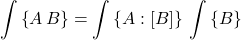(12)(13)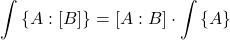## Sums for global climate analysis

### Typical summing

In my analyses of climate, I’ll use the notationto denote a sum over the surface of the globe over some time window:

(14)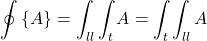where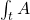is the average over a time window, and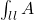is the average over latitude and longitude.

### Time sum

When dealing with climate data, data is usually summed or averaged over some time window, typically at least over a day, if not over a month or a year.

A sum over time can be defined relative to a series of discrete time windows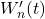or relative to a sliding time window,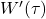.

#### Time sum over a discrete time window

The average of a variable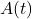relative to a discrete time windowis defined as:

(15)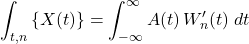where the time window is 1 within the time window, and 0 outside it.

#### Time sum over a sliding time window

The sum of a variablerelative to a sliding time windowis defined as:

(16)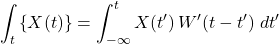where again the time window is 1 within the time window, and 0 outside it.

### Sum over latitude and longitude

The sum ofover latitude and longitude is made up of the integrals over those two variables:

(17)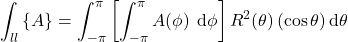## Relationship between sums and averages

Sums and averages, as I’ve defined them, are related as follows:

(18)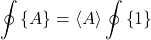(19)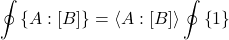The quantity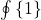is simply the surface area of the planet times the duration of the time window.

Thus, global sums and averages are identical except for a multiplicative constant.